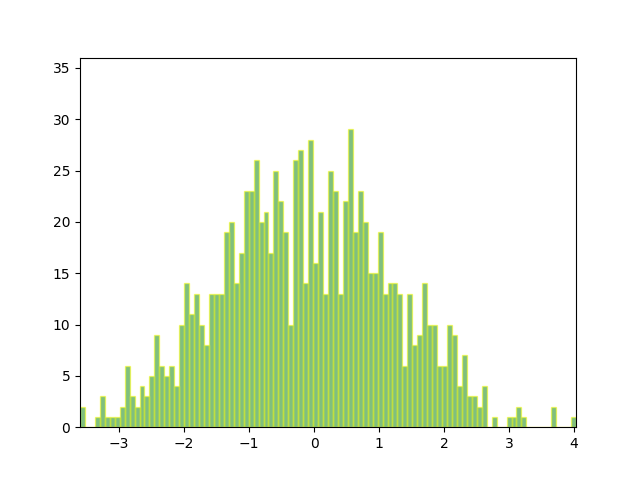You are reading an old version of the documentation (v3.0.0). For the latest version see https://matplotlib.org/stable/gallery/animation/animated_histogram.html

# Animated histogram¶

Use a path patch to draw a bunch of rectangles for an animated histogram.

```import numpy as np

import matplotlib.pyplot as plt
import matplotlib.patches as patches
import matplotlib.path as path
import matplotlib.animation as animation

# Fixing random state for reproducibility
np.random.seed(19680801)

# histogram our data with numpy
data = np.random.randn(1000)
n, bins = np.histogram(data, 100)

# get the corners of the rectangles for the histogram
left = np.array(bins[:-1])
right = np.array(bins[1:])
bottom = np.zeros(len(left))
top = bottom + n
nrects = len(left)
```

Here comes the tricky part -- we have to set up the vertex and path codes arrays using `plt.Path.MOVETO`, `plt.Path.LINETO` and `plt.Path.CLOSEPOLY` for each rect.

• We need 1 `MOVETO` per rectangle, which sets the initial point.
• We need 3 `LINETO`'s, which tell Matplotlib to draw lines from vertex 1 to vertex 2, v2 to v3, and v3 to v4.
• We then need one `CLOSEPOLY` which tells Matplotlib to draw a line from the v4 to our initial vertex (the `MOVETO` vertex), in order to close the polygon.

Note

The vertex for `CLOSEPOLY` is ignored, but we still need a placeholder in the `verts` array to keep the codes aligned with the vertices.

```nverts = nrects * (1 + 3 + 1)
verts = np.zeros((nverts, 2))
codes = np.ones(nverts, int) * path.Path.LINETO
codes[0::5] = path.Path.MOVETO
codes[4::5] = path.Path.CLOSEPOLY
verts[0::5, 0] = left
verts[0::5, 1] = bottom
verts[1::5, 0] = left
verts[1::5, 1] = top
verts[2::5, 0] = right
verts[2::5, 1] = top
verts[3::5, 0] = right
verts[3::5, 1] = bottom
```

To animate the histogram, we need an `animate` function, which generates a random set of numbers and updates the locations of the vertices for the histogram (in this case, only the heights of each rectangle). `patch` will eventually be a `Patch` object.

```patch = None

def animate(i):
# simulate new data coming in
data = np.random.randn(1000)
n, bins = np.histogram(data, 100)
top = bottom + n
verts[1::5, 1] = top
verts[2::5, 1] = top
return [patch, ]
```

And now we build the `Path` and `Patch` instances for the histogram using our vertices and codes. We add the patch to the `Axes` instance, and setup the `FuncAnimation` with our animate function.

```fig, ax = plt.subplots()
barpath = path.Path(verts, codes)
patch = patches.PathPatch(
barpath, facecolor='green', edgecolor='yellow', alpha=0.5)
ax.add_patch(patch)

ax.set_xlim(left, right[-1])
ax.set_ylim(bottom.min(), top.max())

ani = animation.FuncAnimation(fig, animate, 100, repeat=False, blit=True)
plt.show()
```Keywords: matplotlib code example, codex, python plot, pyplot Gallery generated by Sphinx-Gallery